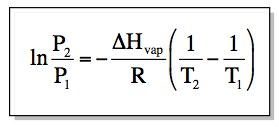Chemistry Practice Problems Clausius-Clapeyron Equation Practice Problems Solution: Butane is a common fuel used in cigarette lighters...

🤓 Based on our data, we think this question is relevant for Professor Aronskyy's class at BARUCH CUNY.

# Solution: Butane is a common fuel used in cigarette lighters and camping stoves. Normally supplied in metal containers under pressure, the fuel exists as a mixture of liquid and gas, so high temperatures may cause the container to explode. At 25.0°C, the vapor pressure of butane is 2.3 atm. What is the pressure in the container at 135°C (ΔH°vap = 24.3 kJ/mol)?

###### Problem

Butane is a common fuel used in cigarette lighters and camping stoves. Normally supplied in metal containers under pressure, the fuel exists as a mixture of liquid and gas, so high temperatures may cause the container to explode. At 25.0°C, the vapor pressure of butane is 2.3 atm. What is the pressure in the container at 135°C (ΔH°vap = 24.3 kJ/mol)?

###### Solution

We can directly determine the vapor pressure of a certain molecule in a particular temperature using the Clausius-Clapeyron equation:View Complete Written Solution

Clausius-Clapeyron Equation

Clausius-Clapeyron Equation

#### Q. Methane (CH4) has a boiling point of −164°C at 1 atm and a vapor pressure of 42.8 atm at −100°C. What is the heat of vaporization of CH4?

Solved • Mon Oct 29 2018 15:00:33 GMT-0400 (EDT)

Clausius-Clapeyron Equation

#### Q. What is the ΔH°vap of a liquid that has a vapor pressure of 621 torr at 85.2°C and a boiling point of 95.6°C at 1 atm?

Solved • Mon Oct 29 2018 14:57:23 GMT-0400 (EDT)

Clausius-Clapeyron Equation

#### Q. Diethyl ether has a ΔH°vap of 29.1 kJ/mol and a vapor pressure of 0.703 atm at 25.0°C. What is its vapor pressure at 95.0°C?

Solved • Mon Oct 29 2018 14:55:38 GMT-0400 (EDT)

Clausius-Clapeyron Equation

#### Q. A liquid has a ΔH°vap of 35.5 kJ/mol and a boiling point of 122°C at 1.00 atm. What is its vapor pressure at 113°C?

Solved • Mon Oct 29 2018 14:53:52 GMT-0400 (EDT)